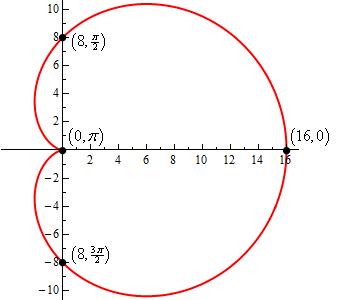Paul's Online Notes
Home / Calculus II / Parametric Equations and Polar Coordinates / Polar Coordinates
Show Mobile Notice Show All Notes Hide All Notes
Mobile Notice
You appear to be on a device with a "narrow" screen width (i.e. you are probably on a mobile phone). Due to the nature of the mathematics on this site it is best views in landscape mode. If your device is not in landscape mode many of the equations will run off the side of your device (should be able to scroll to see them) and some of the menu items will be cut off due to the narrow screen width.

### Section 9.6 : Polar Coordinates

14. Sketch the graph of the following polar equation.

$r = 8 + 8\cos \theta$ Show Solution

We know from the notes on this section that this is a cardioid and so all we really need to get the graph is a quick chart of points.

$$\theta$$ $$r$$ $$\left( {r,\theta } \right)$$
0 16 $$\left( {16,0} \right)$$
$$\displaystyle \frac{\pi }{2}$$ 8 $$\left( {8,\displaystyle \frac{\pi }{2}} \right)$$
$$\pi$$ 0 $$\left( {0,\pi } \right)$$
$$\displaystyle \frac{{3\pi }}{2}$$ 8 $$\left( {8,\displaystyle \frac{{3\pi }}{2}} \right)$$
$$2\pi$$ 16 $$\left( {16,2\pi } \right)$$

So here is the graph of this function.Be careful when plotting these points and remember the rules for graphing polar coordinates. The “tick marks” on the graph are really the Cartesian coordinate tick marks because those are the ones we are familiar with. Do not let them confuse you when you go to plot the polar points for our sketch.